May 18, 2017

# Inferences from Two Samples — practice test 9B

20 cards
Problems with solutions in Elementary Statistics — Inferences About Two Proportions; Inferences About Two Means: Independent Samples; Inferences from Matched Pairs; Comparing Variation in Two Samples.

Directions:

• Provide an appropriate response.
• 1. The test statistic for testing hypothesis about two variances is F = s12/s22 where s12 > s22. Describe the numeric possibilities for this test statistic. Explain the circumstances under which the conclusion would be either that the variances are equal or that the variances are not equal.

The value for the test statistic F will be 1 or greater. If the value is reasonable close to 1, the conclusion is that the two variances are equal. If the value is significantly greater than 1, the conclusion is that the two variances are not equal.

• Find the number of successes x suggested by the given statement.
• 2. Among 730 people selected randomly from among the eligible voters in one city, 64% were homeowners.
1. 467
2. 463
3. 472
4. 471

• From the sample statistics, find the value of p-bar used to test the hypothesis that the population proportions are equal.
• 3. n1 = 216, x1 = 76; n2 = 186, x2 = 99
1. 0.435
2. 0.218
3. 0.392
4. 0.305

• Compute the test statistic used to test the null hypothesis that p1 = p2.
• 4. Information about movie ticket sales was printed in a movie magazine. Out of fifty PG-rated movies, 44% had ticket sales in excess of \$3,000,000. Out of thirty-five R-rated movies, 22% grossed over \$3,000,000.
1. 6.491
2. 3.350
3. 2.089
4. 4.188

• Find the appropriate p-value to test the null hypothesis, H0: p1 = p2, using a significance level of 0.05.
• 5. n1 = 200, x1 = 11; n2 = 100, x2 = 8
1. .1011
2. .4010
3. .0201
4. .0012

• Use the traditional method to test the given hypothesis. Assume that the samples are independent and that they have been randomly selected.
• 6. Use the given sample data to test the claim p1 < p2. Use a significance level of 0.10.
Sample 1: n1 = 462, x1 = 84
Sample 2: n2 = 380, x2 = 95

H0: p1 = p2, H1: p1 < p2.
Test statistic: z = 2.41.
Critical value: z = 1.28.
Reject the null hypothesis. There is sufficient evidence to support the claim that p1 < p2.

1. 7. In a random sample of 360 women, 65% favored stricter gun control laws. In a random sample of 220 men, 60% favored stricter gun control laws. Test the claim that the proportion of women favoring stricter gun control is higher than the proportion of men favoring stricter gun control. Use a significance level of 0.05.

H0: p1 = p2, H1: p1 > p2.
Test statistic: z = 1.21.
Critical values: z = 1.645.
Fail to reject the null hypothesis. There is not sufficient evidence to support the claim that the proportion of women favoring stricter gun control is higher than the proportion of men favoring stricter gun control.

2. Construct the indicated confidence interval for the difference between population proportions p1 — p2. Assume that the samples are independent and that they have been randomly selected.
• 8. x1 = 36, n1 = 80 and x2 = 44, n2 = 85. Construct a 95% confidence interval for the difference between population proportions p1 — p2.
1. 0.269 < p1 — p2 < 0.631
2. –0.220 < p1 — p2 < 0.085
3. 0.298 < p1 — p2 < 0.602
4. –0.249 < p1 — p2 < 0.631

3. Determine whether the samples are independent or consist of matched pair.
• 9. The effect of caffeine as an ingredient is tested with a sample of regular soda and another sample with decaffeinated soda.
1. Matched pairs.
2. Independent samples.

4. Test the indicated claim about the means of two populations. Assume that the two samples are independent and that they have been randomly selected.
• 10. A researcher wishes to determine whether people with high blood pressure can reduce their blood pressure by following a particular diet. Use the sample data below to test the claim that the treatment population mean µ1 is smaller than the control population mean µ2. Test the claim using a significance level of 0.01.
Treatment Group: n1 = 101, x̅1 = 120.5, s1 = 17.4;
Control Group: n2 = 105, x̅2 = 149.3, s2 = 30.2.

H0: µ1 = µ2, H1: µ1 < µ2.
Test statistic t = –8.426.
Critical values: t = –2.364.
Reject the null hypothesis. There is sufficient evidence to support the claim that the treatment population mean µ1 is smaller than the control population µ2. On average, the diet appears effective in lowering blood pressure.

• Construct the indicated confidence interval for the difference between the two population means. Assume that the two samples are independent and that they have been randomly selected.
• 11. Independent samples from two different populations yield the following data: x̅1 = 200, x̅2 = 963, s1 = 19, s2 = 86. The sample size is 433 for both samples. Find the 90 percent confidence interval for µ1 – µ2.
1. –777 < µ1 – µ2 < –749
2. –763 < µ1 – µ2 < –763
3. –770 < µ1 – µ2 < –756
4. –768 < µ1 – µ2 < –758

• Use the computer display to solve the problem.
• 12. When testing for a difference between the means of a treatment group and a placebo group, the computer display below is obtained. Using a 0.01 significance level, is there sufficient evidence to support the claim that the treatment group (variable 1) comes from a population with a mean that is greater than the mean for the placebo population? Explain.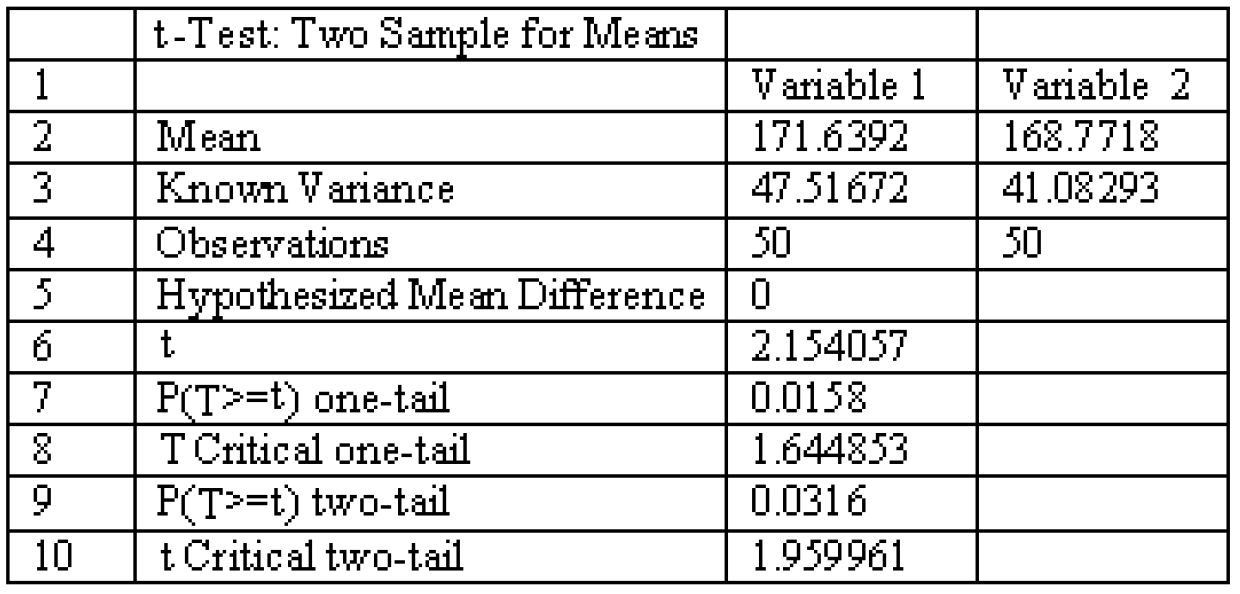No, the P-value for a one-tail test is 0.0158, which is larger than the significant level of 0.01. There is not sufficient evidence to support the claim that the mean for the treatment group is greater than the mean for the placebo group.

• The two data sets are dependent. Find the mean value of the differences d-bar for the paired sample data (d-bar) to the nearest tenth.
• 13. ***
X: 223, 196, 220, 182, 278, 298, 302
Y: 205, 140, 195, 153, 235, 247, 284
1. 34.3
2. 44.6
3. 205.8
4. 20.6

• Find sd.
• 14. The differences between two sets of dependent data are –8, –9, –9, –6, –7. Round to the nearest tenth.
1. 2.6
2. 34.3
3. 1.7
4. 44.6

• Assume that you want to test the claim that the paired sample data come from a population for which the mean difference is µd = 0. Compute the value of the t test statistic.
• 15.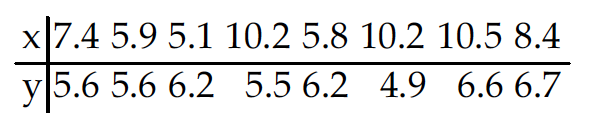1. t = 0.998
2. t = 2.391
3. t = 0.845
4. t = 6.792

• Determine the decision criterion for rejecting the null hypothesis in the given hypothesis test; i.e., describe the values of the test statistic that would result in rejection of the null hypothesis.
• 16. A farmer has decided to use a new additive to grow his crops. He divided his farm into 10 plots and kept records of the corn yield (in bushels) before and after using the additive. The results are shown below.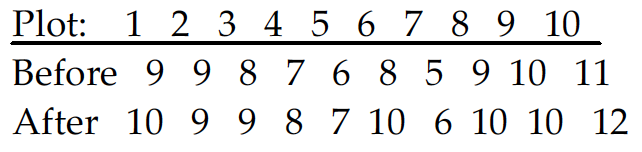You wish the test the following hypothesis at the 1 percent level of significance.
H0: µD = 0 against H1: µD ≠ 0.
What decision rule would you use?
1. Reject H0 if test statistic is greater than 3.250.
2. Reject H0 if test statistic is less than –3.250.
3. Reject H0 if test statistic is less than –3.250 or greater than 3.250.
4. Reject H0 if test statistic is greater than –3.250 or less than 3.250.

• Use the traditional method of hypothesis testing to test the given claim about the means of two populations. Assume that two dependent samples have been randomly selected from normally distributed populations.
• 17. A coach uses a new technique to train gymnasts. 7 gymnasts were randomly selected and their competition scores were recorded before and after the training. The results are shown below: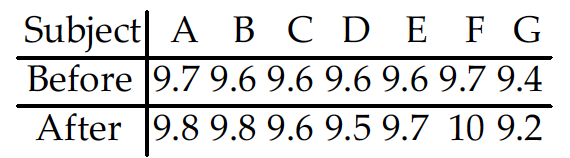Using a 0.01 level of significance, test the claim that the training technique is effective in raising the gymnasts’ scores.

Test statistic t = 0.880.
Critical values: t = –3.143.
Fail to reject H0: µd = 0. There is not sufficient evidence to support the claim that the technique is effective in raising the gymnasts’ scores.

• Construct a confidence interval for µd, the mean of the differences d for the population of paired data. Assume that the population of paired differences is normally distributed.
• 18. Using the sample paired data below, construct a 90% confidence interval for the population mean of all differences x – y.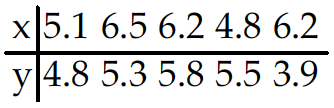1. 0.22 < µd < 7.48
2. –0.07 < µd < 1.47
3. –0.37 < µd < 1.77
4. –0.31 < µd < 1.71

• Test the indicated claim about the variances or standard deviations of two populations. Assume that the populations are normally distributed. Assume that the two samples are independent and that they have been randomly selected.
• 19. Test the claim that populations A and B have different variances. Use a significance level of 0.10.

Sample A: n = 28, x̅ = 19.2, s = 5.38
Sample B: n = 41, x̅ = 23.7, s = 5.89